# Controlling WS2812 Tricolor LEDs (aka NeoPixels), Part 2Controlling WS2812 Tricolor LEDs (aka NeoPixels), Part 2

Turning a LED on is easy. Preventing it from blowing itself up is a little harder.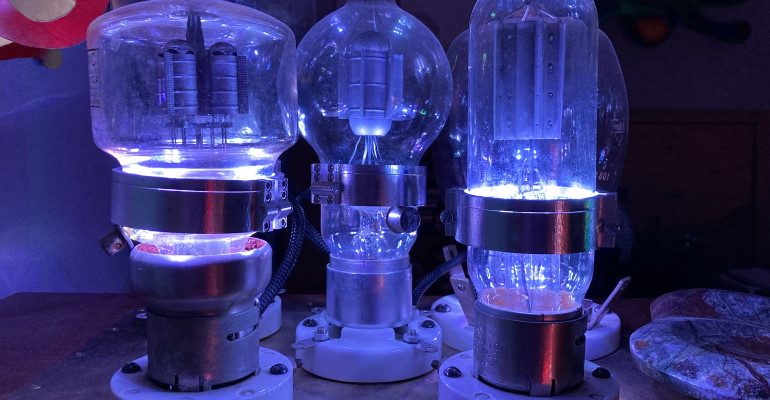Image courtesy of Clive "Max" Maxfield

In my previous column, we covered quite a lot of ground, starting with the first vacuum tube diodes, moving to their solid-state (semiconductor) descendants, and progressing to their light-emitting cousins. We also considered the weighty matter of pertinent pronunciation regarding which is more correct to say, “a LED” or “an LED.”

Now the time has come to delve a little deeper into this fascinating topic. In the case of a discrete (individually packaged) LED, most of the time, we are going to need to include some form of current-limiting resistor in our circuit. But, before we go there, we first need to consider the LED’s forward voltage drop (VF).

I found the concept of forward voltage drop a bit hard to wrap my brain around when I started out deep in the mists of time, but I’ve since come up with what I believe to be a useful analogy. Suppose we are powering our LED circuit with a 5-volt (5V) supply. Let’s visualize this as a river whose water is 5 ft deep. In this case, if you happened to find yourself standing in the river, you would have to struggle against the pressure of a 5-ft wall of water pushing against you (frowny face).

Now, suppose we build a barrier across the riverbed, where this barrier is 2 ft high. In this case, if you decided to hang out in the river downstream of the barrier, you would have only a 3-ft wall of water with which to contend (smiley face). Well, a LED is just the same (but different).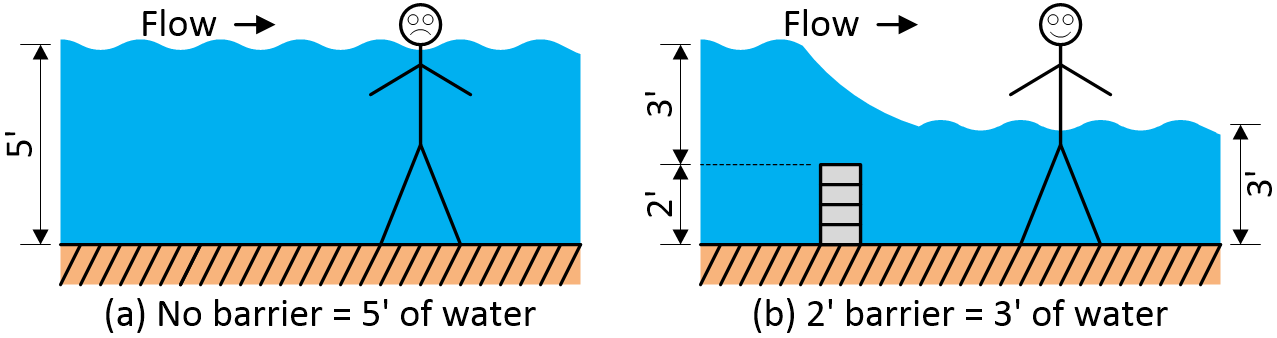Visualizing a LED’s forward voltage drop in terms of water (Image courtesy of Clive

In the case of a LED, when we apply an electrical potential across its terminals, nothing is going to happen until this potential exceeds the LED’s forward voltage drop. What this means is that if the LED’s data sheet gives a VF value of 2V, and if our power supply (VS) is 5V, then once we’ve overcome the forward voltage drop, we have 3V left to play with, as it were.

Another way of looking at things is that when the supply voltage is less than VF, the LED doesn’t conduct and has infinite resistance. However, we are faced with a new problem once the supply voltage exceeds VF and the LED starts to conduct because its resistance falls to close to zero. From Ohm’s law we know that V = I × R, so I = V/R. This means that, as the resistance approaches 0, the current will trend towards infinity. In turn, this means that the LED will glow very brightly for a very short period, after which it will glow no more (sad face).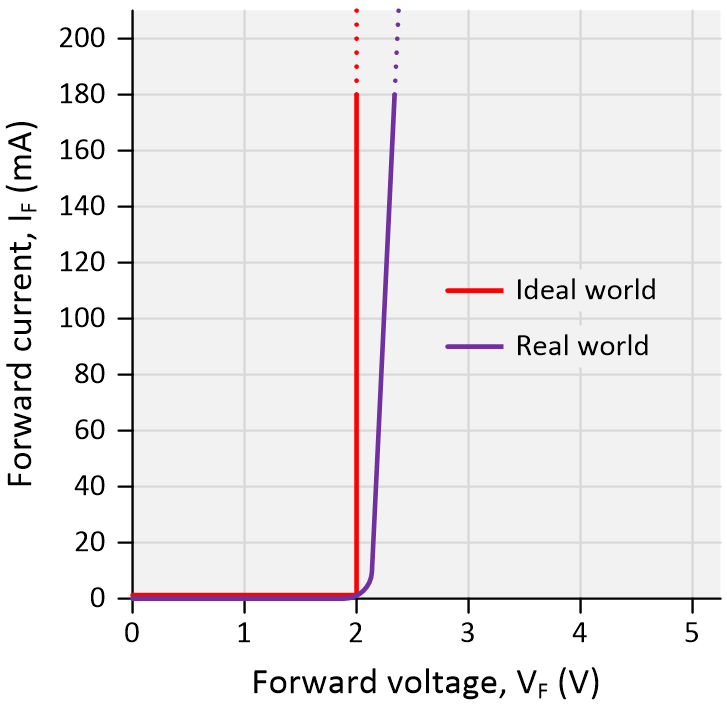Example voltage-current (IV) curves for a generic LED.

This leads us to the other parameter of interest, which is the LED’s maximum permissible forward current (IF). Let’s assume our datasheet specifies this as being 20 mA. Based on this information, we can calculate the value we need to use for our current-limiting resistor (observe that, for our purposes here, the relative positions of the resistor and the LED in the circuit don’t matter).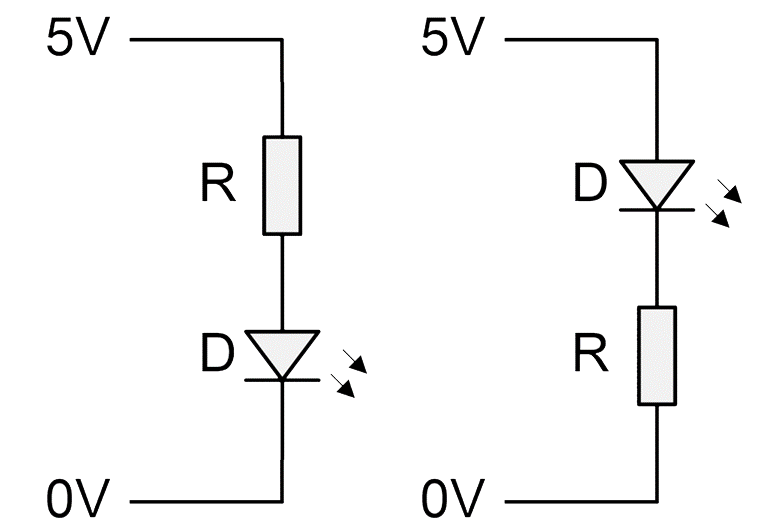The resistor-LED order doesn’t matter.

Once again, from Ohm’s law we know that V = I × R

So, R = V/I

So, R = (Vs – VF)/IF

So, R = (5V – 2V)/20mA

So, R = (3V)/0.02A*

So, R = 150Ω

*Ohm’s law requires that the voltage, current, and resistance are specified in units of volts, amps, and ohms, respectively.

Suppose we want to control a LED using a switch (the way our great-great-grandfathers would have done if they had had a LED ... and a switch). One way would be to connect our +ve power supply to the LED’s anode (via a current-limiting resistor), and to use our switch to connect the LED’s cathode to ground.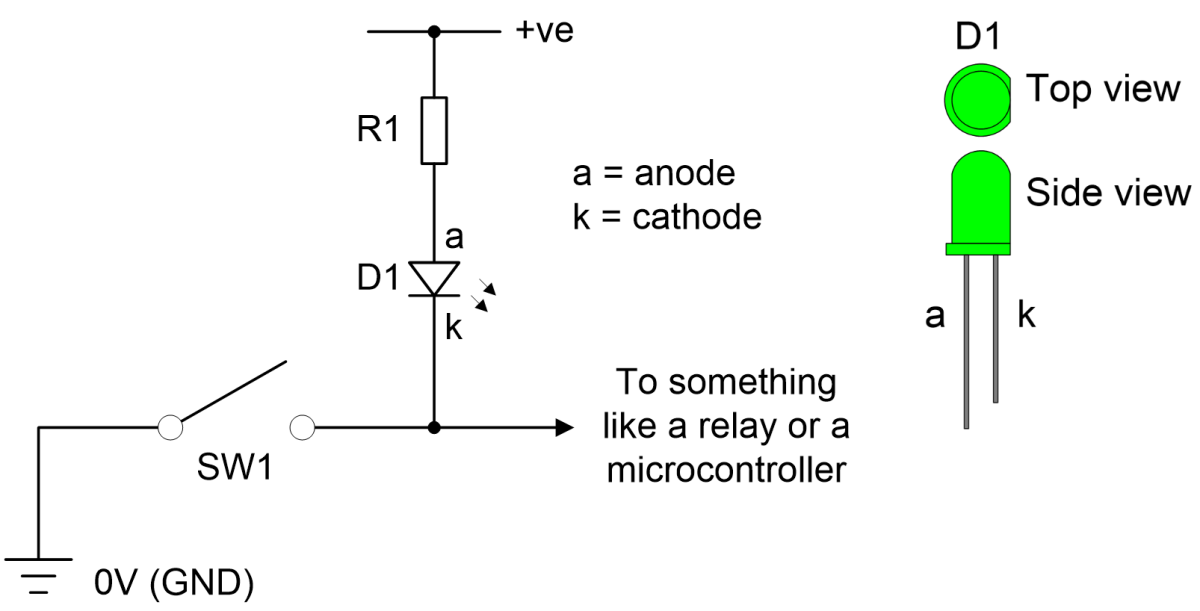One way to control a LED with a switch.

Observe that there are two handy-dandy ways to distinguish the LED’s anode terminal from its cathode – the cathode’s lead is shorter and the side of the package facing the cathode is slightly flattened.

Now let’s suppose that we wish to control our LED using a microcontroller. I’m going to assume an Arduino Uno because that has 5V general-purpose input/outputs (GPIOs) and its programming style is familiar to many of us.

As is usually the case, there are multiple ways in which we can achieve the same end effect. If we connect the cathode side of the LED to ground (as shown in the upper part of the illustration below), then using the microcontroller to drive the pin HIGH (i.e., 5V) will turn the LED on, while driving the pin LOW (i.e., 0V) will turn it off because there will be no electrical potential across its terminals.Two ways to control a LED with a microcontroller.

By comparison, if we connect the anode side of the LED to 5V (as shown in the lower part of the above illustration), then it’s like we are having an “opposite day,” because using the microcontroller to drive the pin HIGH (i.e., 5V) will turn the LED off, while driving the pin LOW (i.e., 0V) will turn it on.

Funnily enough, I got caught out by this myself just the other day as I pen these words. I usually wire my boards as shown in the upper part of the above illustration. On this occasion, however, I was using a board created by someone else to drive three LEDs to display the states of some control switches, and it took me more time than I care to admit before I realized that the reason things were acting wonky was because the board’s designer had wired his LEDs as shown in the lower part of the illustration (there was much gnashing of teeth and rending of garb at that time, let me tell you).

Well, that’s all we’ve got time for at the moment. I know the title of this soon to be mega-mini-series does more than hint that we will be looking at WS2812 Tricolor LEDs (aka NeoPixels), and we will, but laying the foundation before plunging deep into the fray will serve us well as we proceed. As always, I welcome your comments, questions, and suggestions.